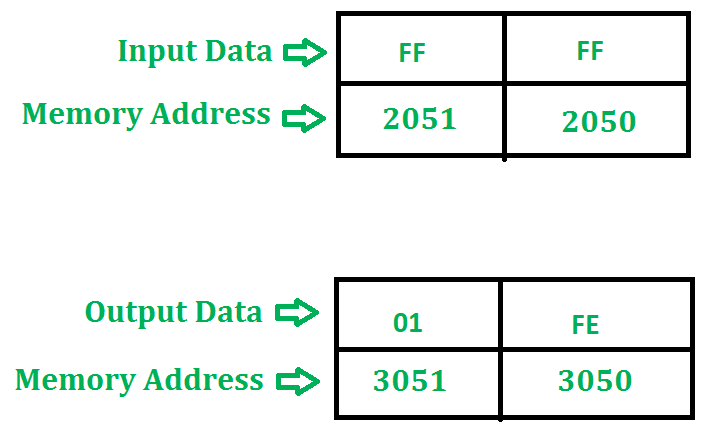GeeksforGeeks App
Open AppBrowser
Continue

# 8085 program to divide two 8 bit numbers

Problem – Write 8085 program to divide two 8 bit numbers.

Example –Algorithm –

2. Move the data to B Register.
3. Load the second data into accumulator.
4. Compare the two numbers to check carry.
5. Subtract two numbers.
6. Increment the value of carry.
7. Check whether the repeated subtraction is over.
8. Then store the results(quotient and remainder) in given memory location.
9. Terminate the program.

Program –

Explanation – Registers A, H, L, C, B are used for general purpose.

1. LXI H, 2050 will load the HL pair register with the address 2050 of memory location.

2. MOV B, M copies the content of memory into register B.

3. MVI C, 00 assign 00 to C.

4. INX H increment register pair HL.

5. MOV A, M copies the content of memory into accumulator.

6. CMP B compares the content of accumulator and register B.

8. SUB B subtract the content of accumulator with register B and store the result in accumulator.

9. INR C increment the register C.

10. JMP 2008 control will shift to memory address 2008.

11. STA 3050 stores the remainder at memory location 3050.

12. MOV A, C copies the content of register into accumulator.

13. STA 3051 stores the quotient at memory location 3051.

14. HLT stops executing the program and halts any further execution.
My Personal Notes arrow_drop_up